# nLab STU relation

Contents

### Context

#### Knot theory

knot theory

Examples/classes:

Types

knot invariants

Related concepts:

category: knot theory

# Contents

## Idea

In knot theory, by the STU-relations, one means the following relations in the linear span of Jacobi diagrams: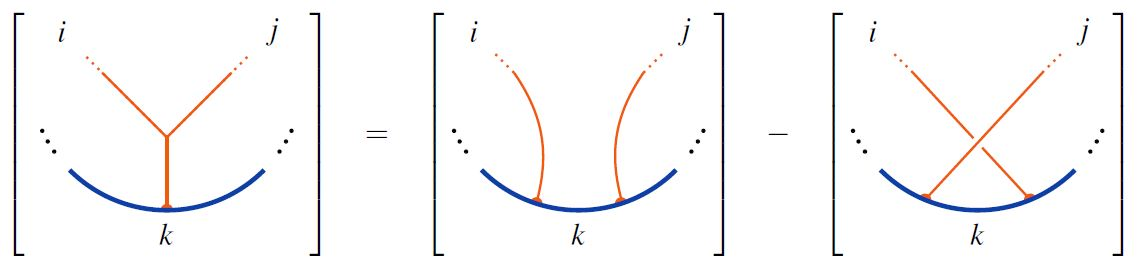graphics from Sati-Schreiber 19c

## Properties

### Relation to weight systems and Lie algebra objects

By definition, the STU-relations are the relations respected by weight systems on Jacobi diagrams.

When regarded as relations of string diagrams, the STU relations characterize the Jacobi identity for Lie algebra objects, or more generally the Lie action property of Lie modules: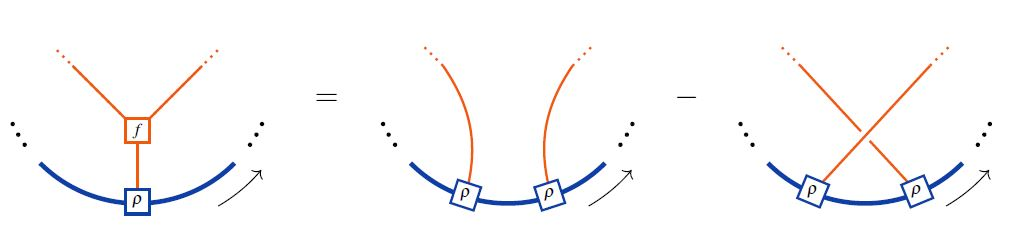\begin{aligned} \Leftrightarrow & \;\;\;\;\; \rho(f(x,y),z) \;=\; \rho(y,\rho(x,z)) - \rho(x,\rho(y,z)) \\ \underset{ {f = [-,-]} \atop {Lie\;bracket} }{ \Leftrightarrow } & \;\;\;\;\; \underset{ {Lie\;action\;property} }{ \underbrace{ \rho([x,y],z) \;=\; \rho(y,\rho(x,z)) - \rho(x,\rho(y,z)) } } \\ \underset{ {\rho = -[-,-]} \atop {adjoint\;action} }{ \Leftrightarrow } & \;\;\;\;\; \underset{ {Jacobi\;identity} }{ \underbrace{ [[x,y],z] \;=\; - [y,[x,z]] + [x,[y,z]] } } \end{aligned}

This is the reason behind the existence of Lie algebra weight systems.

### Relation to 4T-relations

Under the embedding of the set of round chord diagrams into the set of Jacobi diagrams, the STU-relations imply the 4T relations on round chord diagrams: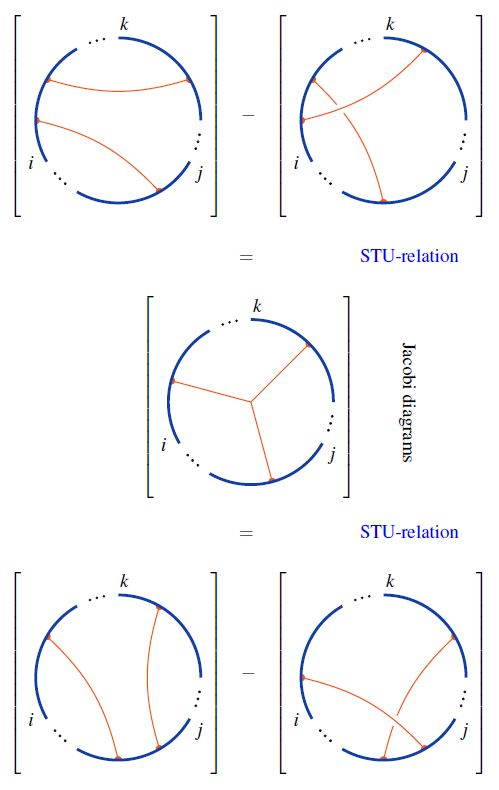Using this, one finds that chord diagrams modulo 4T are Jacobi diagrams modulo STU: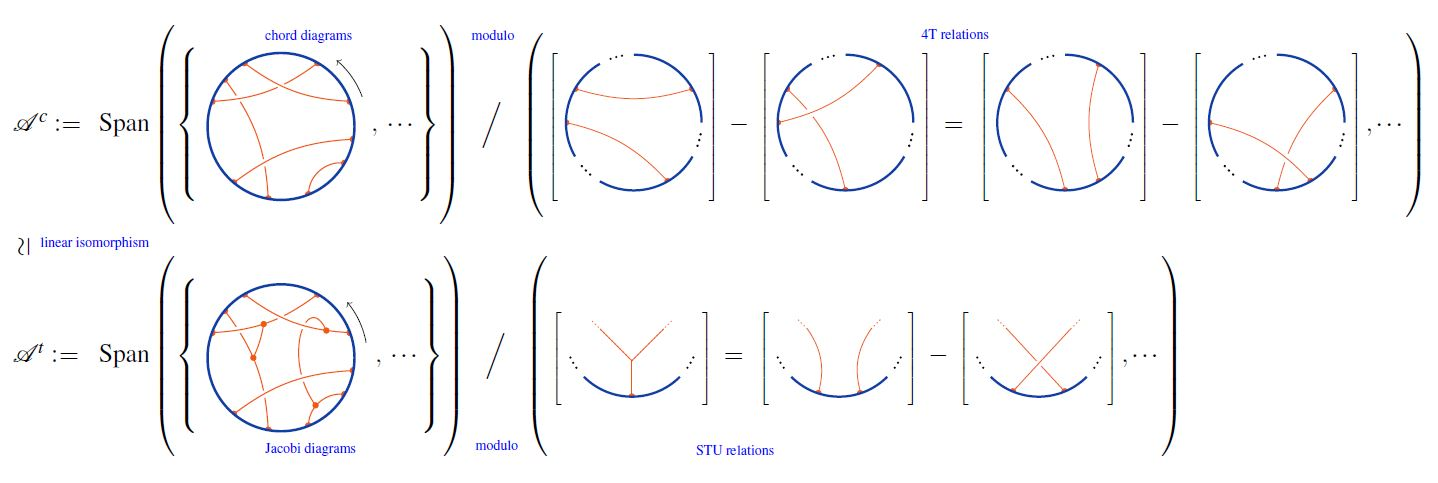graphics from Sati-Schreiber 19c

### Relation to knot-graph complex

That the STU-relations characterize the cochain cohomology of the knot-graph complex in the respective degrees is shown in Koytcheff-Munson-Volic 13, Section 3.4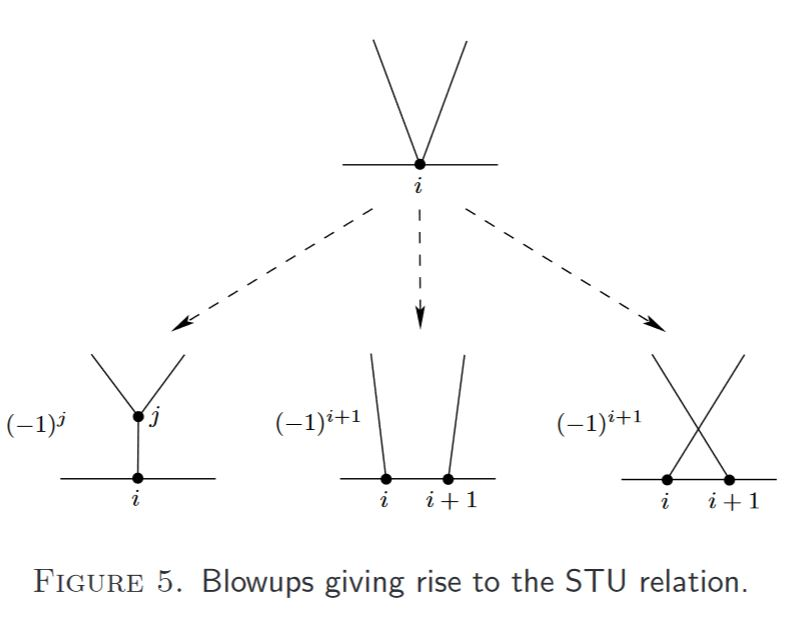graphics grabbed from Koytcheff-Munson-Volic 13

### General

Original articles:

Textbook accounts:

Lecture notes:

### In terms of the knot-graph complex

In terms of the knot-graph complex:

• Robin Koytcheff, Brian A. Munson, Ismar Volić, Section 3.4 of: Configuration space integrals and the cohomology of the space of homotopy string links, J. Knot Theory Ramif. 22, no. 11, 73 pp. (2013) (arXiv:1109.0056)